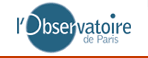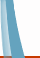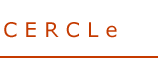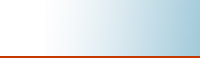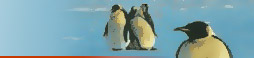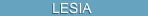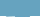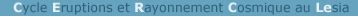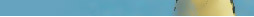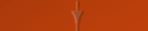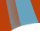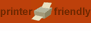[INTRODUCTION]      [PRECURSORS]

How to predict the solar cycle ?

The methods to predict the solar cycle are medium-term methods and long-term methods.

The medium-term methods predict the time profile of the cycle a few months in advance. They are in fact extrapolations of the last sunspot index RI12 observations, taking into account the time profiles of the past cycles. The predictions are generally restricted to about six months because the time profile is difficult to extrapolate, in particular at the beginning of the cycle. A method frequently used for operational purposes has been proposed by McNish and Lincoln. The prediction is based on the average solar cycle profile and is corrected according to the last RI12 observation.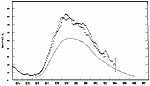Next figure (from Coffey, 1986) shows the mean cycle, the observed cycle (dots) and the prediction one year ahead (small open circles). The predictions presented here use this method. Another medium-term method uses artificial neural networks.

The long-term prediction methods are mostly based on precursors. The precursors are related to measurements on the sun (polar faculae or coronal holes for example), to characteristics of the time profile of the cycle or to geomagnetic activity. We have selected 5 precursors of each of the last two classes.
The precursor methods are simply obtained by application of a linear regression between the precursor (independent variable) and RImax (dependent variable). The statistics involved all the cycles for which the precursor is known. The efficiency of a method could be quantified with the regression coefficient r: a perfect methods would have r = 1 or r = -1. If the variables have no statistical relation at all the regression coefficient r = 0. A method is among the best if the regression coefficient is above 0.85 or below -0.85. All the methods based on geomagnetic indices and presented here have a regression coefficient r above 0.85.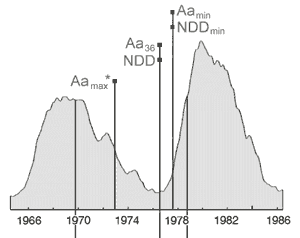The figure illustrates the time at which each of the precursors are available. Precursors based on geomagnetic indices are at the top of the figure. Those based on the time profile of RI12 are below the curves. They are illustrated with pop-up windows. The precursors related to geomagnetic activity are the following :
• Aamax* is the amplitude of a late maximum observed on aa index time profile during the descending phase of the cycle.
• Aa36 is the mean aa index on the three last years of a cycle.
• NDD is the number of disturbed days of the previous cycle (a day is considered as disturbed if the Ap index is higher than 25).
• NDDmin is the minimum of the NDD per month.
• Aamin is the minimum value of the aa index (generally occuring about 1 year after the RI12 minimum).
A recent work (Lantos, 2006) has shown that the McNish and Lincoln method is improved when used in combination with precursors. Indeed in addition to the possibility to know the time profile of a cycle N+1 before its beginning, the precursors limit the errors done with McNish and Lincoln method at the beginning of a cycle, a period were the time profile of the new cycle is not well established.

You can find a more complete description of precursors and methods in the following articles (available in PDF through the "publications" section of this website) :
• McNish & lincoln and neural networks: Fessant F., Pierret C. and Lantos P. Comparison of neural network and McNish and Lincoln methods for the prediction of the smoothed sunspot index. Solar Physics 1996, n°168, pp. 423-433
• Aamax*, Aamin, Aa36 and NDD: Lantos P. and Richard O. On the prediction of maximum amplitude for solar cycles using geomagnetic precursors. Solar Physics 1998, n°182, pp. 231-246
• NDDmin and skewness: Lantos P. Solar cycle prediction: combining precursor methods with McNish and Lincoln technique.
• Inflexion point: Lantos P. Prediction of the maximum amplitude of solar cycles using the ascending inflexion point. Solar Physics 2000, n°196, pp. 221-225